메뉴 건너뛰기

2017.11.11 15:32

조회 수:695

# Plot for distribution of common statistics and p-value

#### 2017-11-11

To understand the concept of p value is very important. To teach the the distribution of common statistic( ${\chi }^{2}$ for chisq.test() , t for Student’s t-test , F for F-test) and concept of the p-value, plot.htest() function can be used.

## Package Installation

You can install this package form the github. Currently, package webr is under construction and consists of only one function - plot.htest().

#install.packages("devtools")
devtools::install_github("cardiomoon/webr")

## Coverage of plot.htest()

The plot.htest() function is a S3 method for class “htest”. Currently, this function covers Welch Two Sample t-test, Pearson’s Chi-squared test, Two Sample t-test, One Sample t-test, Paired t-test and F test to compare two variances.

## For Chi-squared Test

You can show the distribution of chi-squre statistic and p-value.

 require(moonBook)
require(webr)

# chi-squared test
x=chisq.test(table(acs$sex,acs$DM))
x

Pearson's Chi-squared test with Yates' continuity correction

data:  table(acs$sex, acs$DM)
X-squared = 3.1296, df = 1, p-value = 0.07688
 plot(x)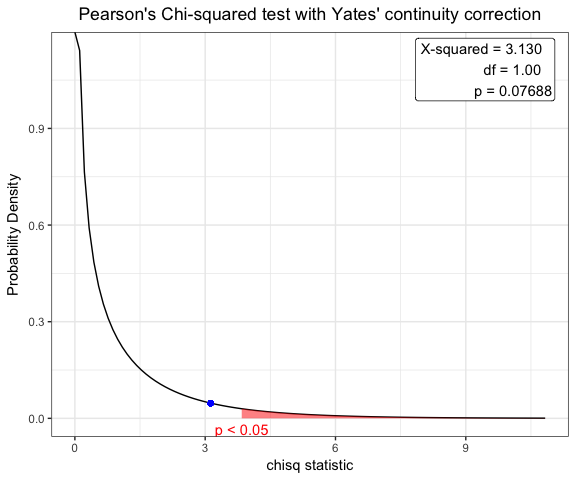## For one sample t-test

You can show the distribution of t-statistic and p-value in one sample t-test.

t.test(acs$age,mu=63)  One Sample t-test data: acs$age
t = 0.77978, df = 856, p-value = 0.4357
alternative hypothesis: true mean is not equal to 63
95 percent confidence interval:
62.52736 64.09574
sample estimates:
mean of x
63.31155 
plot(t.test(acs$age,mu=63))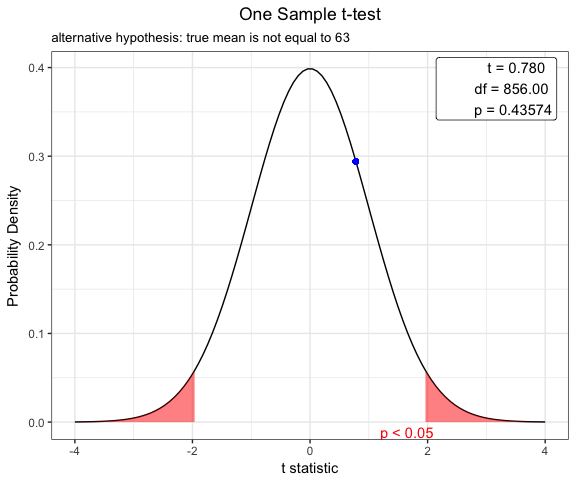## Student t-test to compare means for two independent samples Before performing a t-test, you have to compare two variances. ### F test to compare two variances x=var.test(age~DM,data=acs) x  F test to compare two variances data: age by DM F = 1.2383, num df = 552, denom df = 303, p-value = 0.0376 alternative hypothesis: true ratio of variances is not equal to 1 95 percent confidence interval: 1.012416 1.505776 sample estimates: ratio of variances 1.238288  plot(x)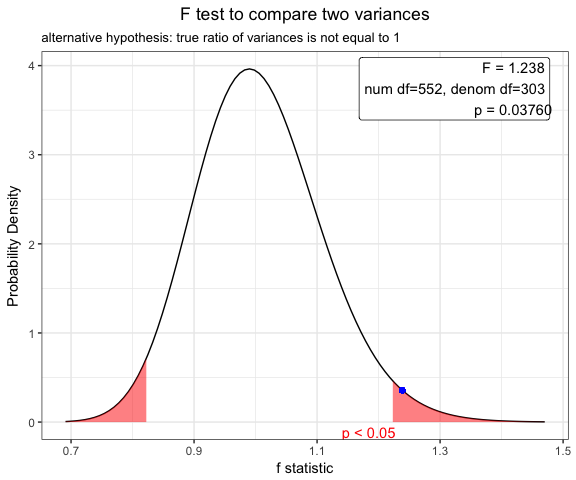### Use for Two Sample t-test for independence samples Based on the result of var.test(), you can perform t.test with default option(var.equal=FALSE). x=t.test(age~DM,data=acs) x  Welch Two Sample t-test data: age by DM t = 0.58982, df = 682.36, p-value = 0.5555 alternative hypothesis: true difference in means is not equal to 0 95 percent confidence interval: -1.112568 2.068014 sample estimates: mean in group No mean in group Yes 63.48101 63.00329  plot(x)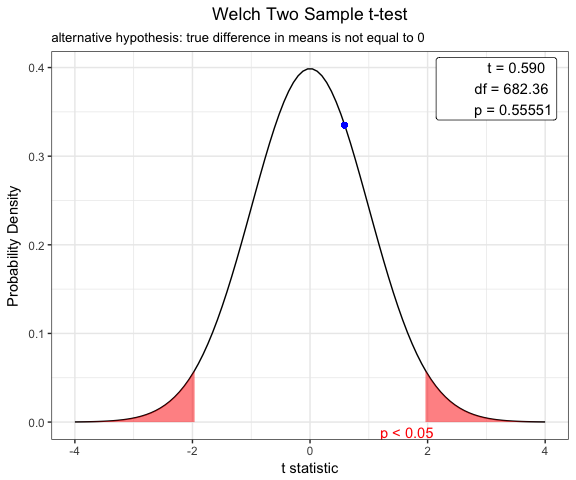## Student t-test using pooled variance To compare means of body-mass index between male and female patients, perform F test first. var.test(BMI~sex,data=acs)  F test to compare two variances data: BMI by sex F = 1.2078, num df = 254, denom df = 508, p-value = 0.07756 alternative hypothesis: true ratio of variances is not equal to 1 95 percent confidence interval: 0.9794315 1.5008098 sample estimates: ratio of variances 1.207759  plot(var.test(BMI~sex,data=acs))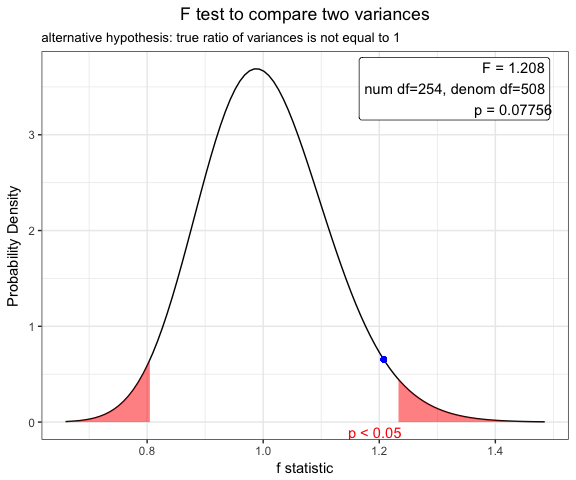Based on the result of F test, you can perform t-test using pooled variance. x=t.test(BMI~sex,data=acs,var.equal=TRUE) x  Two Sample t-test data: BMI by sex t = -0.50823, df = 762, p-value = 0.6114 alternative hypothesis: true difference in means is not equal to 0 95 percent confidence interval: -0.6348532 0.3737344 sample estimates: mean in group Female mean in group Male 24.19492 24.32548  plot(x)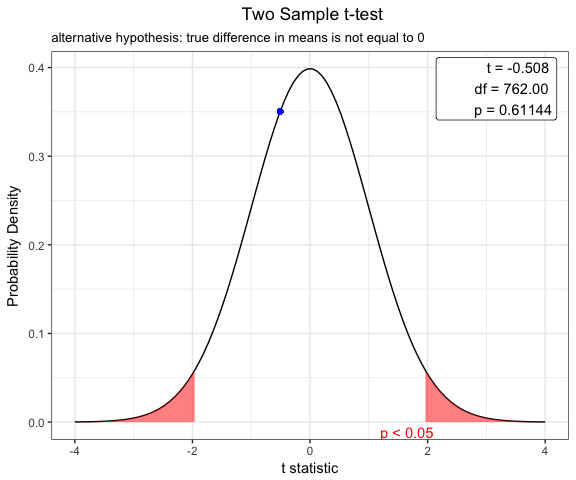## Paired t-test You can show the distribution of t-statistic and p-value in paired t-test. x=t.test(iris$Sepal.Width,iris\$Petal.Width,paired=TRUE)
plot(x)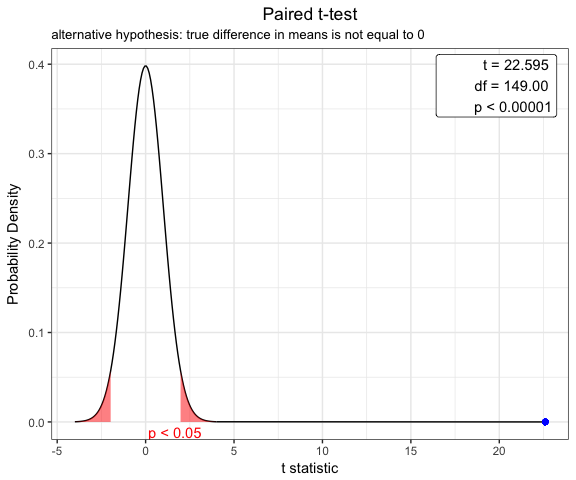## Options for t-test

You can change the options of t.test.

x=t.test(BMI~sex, data=acs,conf.level=0.99,alternative="greater",var.equal=TRUE)
plot(x)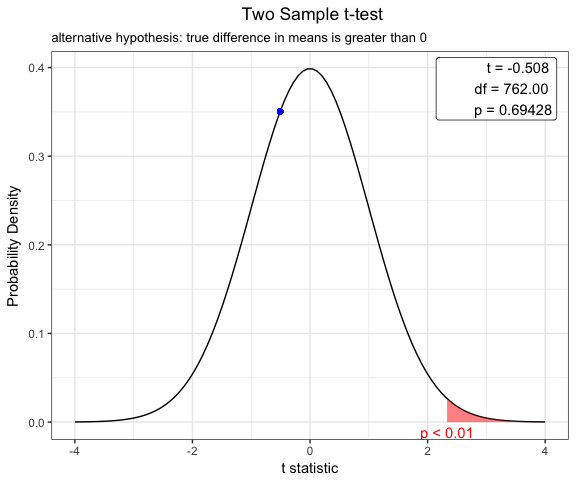번호 제목 글쓴이 날짜 조회 수
공지 웹에서 하는 R 메타분석  2015.11.11 7605
공지 웹에서 하는 R 통계 게시판입니다. 2015.03.26 1619
83 안녕하세요2019.02.27 9
82 moonBook 패키지 업데이트 안내 2018.05.26 699
81 Make a Heatmap Table using ztable 2018.05.23 425
80 moonBook 패키지 업데이트 안내  2018.05.18 3067
79 ztable 패키지 업데이트 안내 2018.05.13 594
78 pptxList: A Shiny Module for Reproducible Research 2018.03.07 682
77 Nightingale's Rose Plot2017.11.16 484
» Plot for distribution of common statistics and p-value 2017.11.11 695
75 R package gglotAssist 2017.11.11 610
74 설문조사데이터 2017.10.14 953
73 R에서 데이터 편집을 하자 - editData 패키지  2017.09.25 2074
72 dplyrAssist 패키지 : RStudio Addin으로 dplyr을 쉽게 배우기  2017.09.03 399
71 "틀리지않는법" 강의슬라이드(문건웅) 2017.08.31 2110
70 연속형변수를 기준으로 같은 크기를 갖는 여러 개의 그룹으로 나누기 2017.06.19 1412
69 3D visualization of multiple regression analysis2017.06.15 446
68 Visualize multiple regression model 2017.06.12 916
67 Longest common substring 2017.05.29 770
66 파일을 업로드 했는데 예제데이터에서 대체가 안됩니다.  2017.05.16 1010
65 샤이니 앱 : interactive ggplot2017.02.28 1987
64 Procedural Programming vs Functional Programming(I) 2017.02.27 172